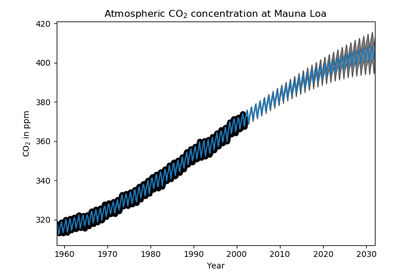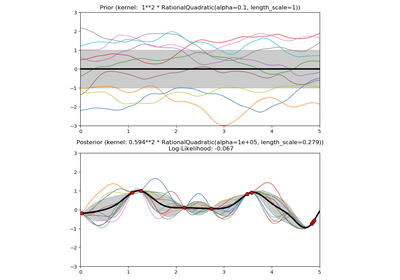# `sklearn.gaussian_process.kernels`.RationalQuadratic¶

class `sklearn.gaussian_process.kernels.``RationalQuadratic`(length_scale=1.0, alpha=1.0, length_scale_bounds=(1e-05, 100000.0), alpha_bounds=(1e-05, 100000.0))[source]

The RationalQuadratic kernel can be seen as a scale mixture (an infinite sum) of RBF kernels with different characteristic length-scales. It is parameterized by a length-scale parameter length_scale>0 and a scale mixture parameter alpha>0. Only the isotropic variant where length_scale is a scalar is supported at the moment. The kernel given by:

k(x_i, x_j) = (1 + d(x_i, x_j)^2 / (2*alpha * length_scale^2))^-alpha

New in version 0.18.

Parameters: length_scale : float > 0, default: 1.0 The length scale of the kernel. alpha : float > 0, default: 1.0 Scale mixture parameter length_scale_bounds : pair of floats >= 0, default: (1e-5, 1e5) The lower and upper bound on length_scale alpha_bounds : pair of floats >= 0, default: (1e-5, 1e5) The lower and upper bound on alpha

Methods

 `clone_with_theta`(theta) Returns a clone of self with given hyperparameters theta. `diag`(X) Returns the diagonal of the kernel k(X, X). `get_params`([deep]) Get parameters of this kernel. `is_stationary`() Returns whether the kernel is stationary. `set_params`(**params) Set the parameters of this kernel.
`__init__`(length_scale=1.0, alpha=1.0, length_scale_bounds=(1e-05, 100000.0), alpha_bounds=(1e-05, 100000.0))[source]
`__call__`(X, Y=None, eval_gradient=False)[source]

Return the kernel k(X, Y) and optionally its gradient.

Parameters: X : array, shape (n_samples_X, n_features) Left argument of the returned kernel k(X, Y) Y : array, shape (n_samples_Y, n_features), (optional, default=None) Right argument of the returned kernel k(X, Y). If None, k(X, X) if evaluated instead. eval_gradient : bool (optional, default=False) Determines whether the gradient with respect to the kernel hyperparameter is determined. Only supported when Y is None. K : array, shape (n_samples_X, n_samples_Y) Kernel k(X, Y) K_gradient : array (opt.), shape (n_samples_X, n_samples_X, n_dims) The gradient of the kernel k(X, X) with respect to the hyperparameter of the kernel. Only returned when eval_gradient is True.
`bounds`

Returns the log-transformed bounds on the theta.

Returns: bounds : array, shape (n_dims, 2) The log-transformed bounds on the kernel’s hyperparameters theta
`clone_with_theta`(theta)[source]

Returns a clone of self with given hyperparameters theta.

`diag`(X)[source]

Returns the diagonal of the kernel k(X, X).

The result of this method is identical to np.diag(self(X)); however, it can be evaluated more efficiently since only the diagonal is evaluated.

Parameters: X : array, shape (n_samples_X, n_features) Left argument of the returned kernel k(X, Y) K_diag : array, shape (n_samples_X,) Diagonal of kernel k(X, X)
`get_params`(deep=True)[source]

Get parameters of this kernel.

Parameters: deep : boolean, optional If True, will return the parameters for this estimator and contained subobjects that are estimators. params : mapping of string to any Parameter names mapped to their values.
`hyperparameters`

Returns a list of all hyperparameter specifications.

`is_stationary`()[source]

Returns whether the kernel is stationary.

`n_dims`

Returns the number of non-fixed hyperparameters of the kernel.

`set_params`(**params)[source]

Set the parameters of this kernel.

The method works on simple kernels as well as on nested kernels. The latter have parameters of the form `<component>__<parameter>` so that it’s possible to update each component of a nested object.

Returns: self :
`theta`

Returns the (flattened, log-transformed) non-fixed hyperparameters.

Note that theta are typically the log-transformed values of the kernel’s hyperparameters as this representation of the search space is more amenable for hyperparameter search, as hyperparameters like length-scales naturally live on a log-scale.

Returns: theta : array, shape (n_dims,) The non-fixed, log-transformed hyperparameters of the kernel

## Examples using `sklearn.gaussian_process.kernels.RationalQuadratic`¶Gaussian process regression (GPR) on Mauna Loa CO2 data.Illustration of prior and posterior Gaussian process for different kernels